# Java Generics Tutorial with Examples

#### View more Tutorials: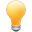Follow us on our fanpages to receive notifications every time there are new articles.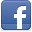Facebook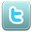Twitter## 1- Why Java Generics?

Generics is a concept which is put into Java since version 5. Before introducing the concept of generics, we see a snippet of Java code prior to version 5.
In this example, ArrayList is a list in which  you can add, delete, modify the list, and access to the elements of the list.
BeforeJ5Example.java
``````
package org.o7planning.tutorial.generics;

import java.util.ArrayList;

public class BeforeJ5Example {

public static void main(String[] args) {

// To contain the names of the users.

// You accidentally added a non-string element to the list.
// (This is allowed).

// And get the first element.
// It is an Object (But you know it is a String)
// ==> Tom

// Cast to String.

// And get the second element.
// (You know it is a String)
// ==> jerry

// Get the 3rd element and cast it to a String.
// (Actually it is an Integer).
// (Error casts happen here).

}

}
``````
A situation in Java older version 5:

You create an ArrayList object for the purpose of only containing elements with the type of String , however, you add to this list an element that not a String type at somewhere in the program (This is entirely possible), while you get out of that element and cast to a String type, an exception will be thrown.
• TODO (Image)
Java 5 put into concept of Generics. With the help of Generics, you can create an ArrayList object which only allows to contain elements with type of String, and not allows to contain elements with other types.
J5Example.java
``````
package org.o7planning.tutorial.generics;

import java.util.ArrayList;

public class J5Example {
public static void main(String[] args) {
// Create an ArrayList.
// This list only allows elements of type String.

// You can not add an element not a String
// (Error compiling).

// You do not need to cast the element.
}
}
``````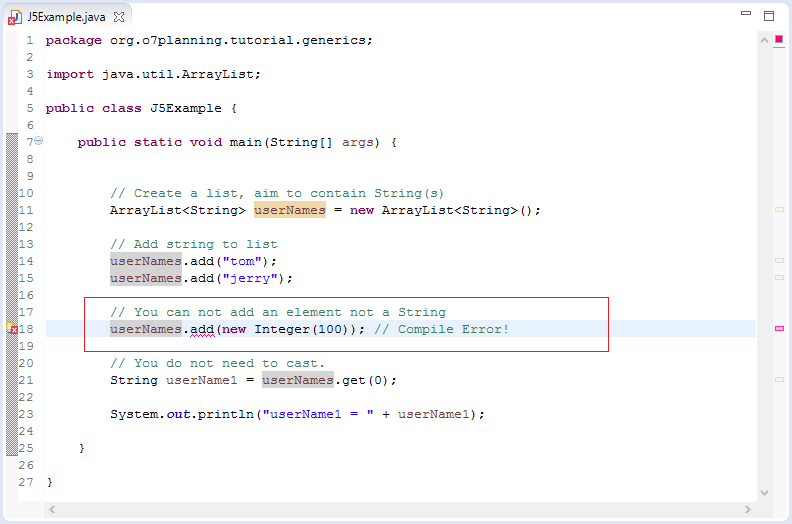When you create an  ArrayList <String> object, it only contains elements with type of String, the Java compiler does not allow this object to contains the elements that are different from String.

## 2- Generic type for Class & Interface

### 2.1- Generics Class

The example below defines a  generics class. KeyValue is a generics class that contains pairs of key and value.
KeyValue.java
``````
package org.o7planning.tutorial.generics.ci;

public class KeyValue<K, V> {

private K key;
private V value;

public KeyValue(K key, V value) {
this.key = key;
this.value = value;
}

public K getKey() {
return key;
}

public void setKey(K key) {
this.key = key;
}

public V getValue() {
return value;
}

public void setValue(V value) {
this.value = value;
}

}
``````
K, V in  KeyValue <K, V> class  is called generics parameter which  is a certain type of reference. When using this class, you must determine the specific parameter.

Take the example of using  KeyValue class
KeyValueDemo.java
``````
package org.o7planning.tutorial.generics.ci;

public class KeyValueDemo {

public static void main(String[] args) {

// Create KeyValue object.
// Integer: Phone Number (K = Integer)
// String: Name of User (V = String)
KeyValue<Integer, String> entry = new KeyValue<Integer, String>(12000111, "Tom");

// Java understands that the return type is a Integer
// (K = Integer)
Integer phone = entry.getKey();

// Java understands that the return type is a String
// (V = String).
String name = entry.getValue();

System.out.println("Phone = " + phone + " / name = " + name);
}

}
``````
Running Example:
``````
Phone = 12000111 / name = Tom
``````

### 2.2- Inheritance Generics class

A class extended from a generics class  can specify parameter type for generics, retain generics parameters  or add  generics parameters .
Example 1:
PhoneNameEntry.java
``````
package org.o7planning.tutorial.generics.ci;

// This class extends KeyValue<K,V>.
// And specify K, V:
// K = Integer (Phone Number)
// V = String (Name)
public class PhoneNameEntry extends KeyValue<Integer, String> {

public PhoneNameEntry(Integer key, String value) {
super(key, value);
}

}
``````
Example use PhoneNameEntry:
PhoneNameEntryDemo.java
``````
package org.o7planning.tutorial.generics.ci;

public class PhoneNameEntryDemo {

public static void main(String[] args) {

PhoneNameEntry entry = new PhoneNameEntry(12000111, "Tom");

// Java understands that the return type is Integer.
Integer phone = entry.getKey();

// Java understands that the return type is String.
String name = entry.getValue();

System.out.println("Phone = " + phone + " / name = " + name);

}

}
``````
Example 2:
StringAndValueEntry.java
``````
package org.o7planning.tutorial.generics.ci;

// This class extends KeyValue<K,V>.
// Specify the parameter <K> is String.
// Stil keep Generics Parameter <V>.
public class StringAndValueEntry<V> extends KeyValue<String, V> {

public StringAndValueEntry(String key, V value) {
super(key, value);
}

}
``````
Example use StringAndValueEntry class:
StringAndValueEntryDemo.java
``````
package org.o7planning.tutorial.generics.ci;

public class StringAndValueEntryDemo {

public static void main(String[] args) {

// (Emp Number, Employee Name)
// V = String (Employee Name)
StringAndValueEntry<String> entry = new StringAndValueEntry<String>("E001", "Tom");

String empNumber = entry.getKey();

String empName = entry.getValue();

System.out.println("Emp Number = " + empNumber);
System.out.println("Emp Name = " + empName);

}

}
``````
Example 3:
KeyValueInfo.java
``````
package org.o7planning.tutorial.generics.ci;

// This class extends from KeyValue<K,V> class.
// It has one more Generics parameter <I>.
public class KeyValueInfo<K, V, I> extends KeyValue<K, V> {

private I info;

public KeyValueInfo(K key, V value) {
super(key, value);
}

public KeyValueInfo(K key, V value, I info) {
super(key, value);
this.info = info;
}

public I getInfo() {
return info;
}

public void setInfo(I info) {
this.info = info;
}

}
``````

### 2.3- Generics Interface

Interface with Generics:
GenericInterface.java
``````
package org.o7planning.tutorial.generics.ci;

public interface GenericInterface<G> {

public G doSomething();

}
``````
For example, an implementation of the interface:
GenericInterfaceImpl.java
``````
package org.o7planning.tutorial.generics.ci;

public class GenericInterfaceImpl<G> implements GenericInterface<G>{

private G something;

@Override
public G doSomething() {
return something;
}

}
``````

### 2.4- Java doesn't support generic Throwable

You can not create a generic class which is a descendant of Throwable, because java does not support to create such class.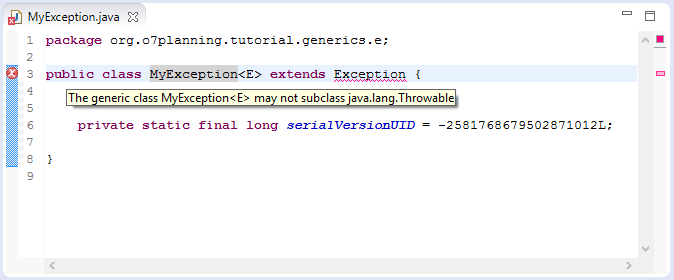Error message of compiler:
``````
- The generic class MyException<E> may not subclass java.lang.Throwable
``````
Java do not support in creating a Throwable generic class because it does not bring any benefit. The reason is that the information is only used for  code control compiler of programmers. In the  runtime of Java, Generic information does not exist, an object of Mistake <Account> or Mistake <User> are an object type of Mistake.
``````
} catch( Mistake<Account> ea) {
// If Mistake exception occurs, this block will be executed.
...
} catch( Mistake<User> eu) {
// This block is never executed
...
}
``````

## 3- Generic Methods

A method in class or Interface may be generified.
MyUtils.java
``````
package org.o7planning.tutorial.generics.m;

import java.util.ArrayList;

import org.o7planning.tutorial.generics.ci.KeyValue;

public class MyUtils {

// <K, V>: To say that this method has two parameters K, V
// This method returns an object of type K.
public static <K, V> K getKey(KeyValue<K, V> entry) {
K key = entry.getKey();
return key;
}

// <K, V>: To say that this method has two parameters K, V
// Method returns an object of type V.
public static <K, V> V getValue(KeyValue<K, V> entry) {
V value = entry.getValue();
return value;
}

// ArrayList <E>: The list contains the elements of type E.
// Method returns an object of type E.
public static <E> E getFirstElement(ArrayList<E> list) {
if (list == null || list.isEmpty()) {
return null;
}
E first = list.get(0);
return first;
}

}
``````
For example, using generics method :
MyUtilsDemo.java
``````
package org.o7planning.tutorial.generics.m;

import java.util.ArrayList;

import org.o7planning.tutorial.generics.ci.KeyValue;

public class MyUtilsDemo {

public static void main(String[] args) {

// K = Integer: Phone
// V = String: Name
KeyValue<Integer, String> entry1 = new KeyValue<Integer, String>(12000111, "Tom");
KeyValue<Integer, String> entry2 = new KeyValue<Integer, String>(12000112, "Jerry");

// (K = Integer).
Integer phone = MyUtils.getKey(entry1);
System.out.println("Phone = " + phone);

// A list containing the element type of KeyValue<Integer, String>.
ArrayList<KeyValue<Integer, String>> list = new ArrayList<KeyValue<Integer, String>>();

KeyValue<Integer, String> firstEntry = MyUtils.getFirstElement(list);

System.out.println("Value = " + firstEntry.getValue());
}

}
``````

## 4- Generic Object Initialization

Sometimes, you want to initialize a Generic object:
``````
// Create a Generic object.
T t = new T(); // Error
``````
Initiating  a generic object as above is not allowed, because <T> does not exist in the runtime of Java. It only means to code control  compiler  of programmers. All types of <T> are  the same and it is understood as Object at the runtime of Java

If you want to initialize  Generic object,  you need to pass Class<T> object to Java which helps Java to create generic object at runtime by using Java Reflection.
Bar.java
``````
package org.o7planning.tutorial.generics.o;

import java.util.Date;

public class Bar {

// This class must have a default constructor.
public Bar() {

}

public void currentDate() {
System.out.println("Now is: " + new Date());
}

}
``````
MyGeneric.java
``````
package org.o7planning.tutorial.generics.o;

public class MyGeneric<T> {

private T tobject;

public MyGeneric(Class<T> tclass)
throws InstantiationException, IllegalAccessException {

this.tobject = (T) tclass.newInstance();

}

public T getTObject() {
return this.tobject;
}
}
``````
MyGenericDemo.java
``````
package org.o7planning.tutorial.generics.o;

public class MyGenericDemo {

public static void main(String[] args) throws Exception {

MyGeneric<Bar> mg = new MyGeneric<Bar>(Bar.class);

Bar bar = mg.getTObject();

bar.currentDate();
}
}
``````

## 5- Generic Array

You can declare a generic array, but you can not initialize a generic array.
``````
// You can declare a generic array.
T[] myarray;

// But you can not initialize a generic array.
// (This is not allowed).
T[] myarray = new T;  // Error!
``````
Example:
GenericArray.java
``````
package org.o7planning.tutorial.generics.a;

public class GenericArray<T> {

private T[] array;

// Contructor.
public GenericArray(T[] array) {
this.array = array;
}

public T[] getArray() {
return array;
}

// Returns the last element of the array.
public T getLastElement() {
if (this.array == null || this.array.length == 0) {
return null;
}
return this.array[this.array.length - 1];
}

}
``````
GenericArrayDemo.java
``````
package org.o7planning.tutorial.generics.a;

public class GenericArrayDemo {

public static void main(String[] args) {

// Array of Strings
String[] names = new String[] { "Tom", "Jerry" };

GenericArray<String> gArray = new GenericArray<String>(names);

String last = gArray.getLastElement();

System.out.println("Last Element = " + last);
}

}
``````
Returning to the question of why Java does not  support to  initialize a Generic array:
``````
// Why Java don't support to initialize Generic array?
T[] genericArray = new T; // Error!
``````
The reason is that generic type does not exist at runtime, List<String> or List<Integer> are List. Generic only works with compilers to check code of programmers. It means that the compiler of Java need to know what is <T> to compile new T ;. Without knowing it will consider T as a Object by default. Then:
``````
// Suppose that Java allows to initialize a Generic array:
T[]  tarray = new T;

// At the time of compilation,
// the compiler will consider <T> as Object.
// The above statement is equivalent to:
T[] tarray  = new Object;

// If at run time of the app you specify <T> as String.
// Mean:
String[] tarray = new Object;

// The above is not allowed. Reason:
// Type mismatch: cannot convert from Object[] to String[]
``````
If you want to initialize  Generic array,  you need to pass Class <T> object to Java which helps Java to create generic array at runtime by using Java Reflection. See examples:
GArray.java
``````
package org.o7planning.tutorial.generics.a;

import java.lang.reflect.Array;

public class GArray<T> {

private Class<T> tclass;

private T[] myArray;

public GArray(Class<T> tclass) {
this.tclass = tclass;

final int size = 10;
myArray = (T[]) Array.newInstance(tclass, size);
}

public T[] getMyArray() {
return this.myArray;
}

}
``````
GArrayDemo.java
``````
package org.o7planning.tutorial.generics.a;

public class GArrayDemo {

public static void main(String[] args) {

GArray<Integer> garray = new GArray<Integer>(Integer.class);

Integer[] myArray = garray.getMyArray();

myArray = 1;
myArray = 0;
}

}
``````

## 6- Generics with Wildcards

In generic code, the question mark (?), called the wildcard, represents an unknown type. A wildcard parameterized type is an instantiation of a generic type where at least one type argument is a wildcard.
Examples of wildcard parameterized types are:
• Collection<?>
• List<? extends Number>
• Comparator<? super String>
• Pair<String,?>.
The wildcard can be used in a variety of situations: as the type of a parameter, field, or local variable; sometimes as a return type (though it is better programming practice to be more specific). The wildcard is never used as a type argument for a generic method invocation, a generic class instance creation, or a supertype.
Having wildcards at difference places have different meanings as well. e.g.
• Collection<?> denotes all instantiations of the Collection interface regardless of the type argument.
• List<? extends Number> denotes all list types where the element type is a subtype of Number.
• Comparator<? super String> denotes all instantiations of the Comparator interface for type argument types that are supertypes of String.
A wildcard parameterized type is not a concrete type that could appear in a new operator. It just hints the rule enforced by java generics that which types are valid in any particular scenario where wild cards have been used.
Example:
``````
Collection<?> coll = new ArrayList<String>();

// A List contains only Number or subtypes of Number.
List<? extends Number> list = new ArrayList<Long>();

// An object with wildcard parameterized type.
Pair<String,?> pair = new Pair<String,Integer>();
``````
Some of the invalid declarations.
``````
// String is not subtype of Number, so error.
List<? extends Number> list = new ArrayList<String>();

// String is not a parent type of Integer, so error.
ArrayList<? super String> cmp = new ArrayList<Integer>();
``````

### 6.1- Examples with wildcard

WildCardExample1.java
``````
package org.o7planning.tutorial.generics.w;

import java.util.ArrayList;

public class WildCardExample1 {

public static void main(String[] args) {

// A list containing the elements of type String.
ArrayList<String> listString = new ArrayList<String>();

// A list containing the elements of type Integer.
ArrayList<Integer> listInteger = new ArrayList<Integer>();

// You can not declare:
ArrayList<Object> list1 = listString; // ==> Error!

// A wildcard parameterized object
ArrayList<? extends Object> list2;

// You can declare:
list2 = listString;

// Or
list2 = listInteger;

}

}
``````
WildCardExample2.java
``````
package org.o7planning.tutorial.generics.w;

import java.util.ArrayList;
import java.util.List;

public class WildCardExample2 {

public static void printElement(List<?> list) {
for (Object e : list) {
System.out.println(e);
}
}

public static void main(String[] args) {

List<String> names = new ArrayList<String>();

List<Integer> values = new ArrayList<Integer>();

System.out.println("--- Names --");

printElement(names);

System.out.println("-- Values --");

printElement(values);

}

}
``````

### 6.2- A wildcard parameterized type can not use generic methods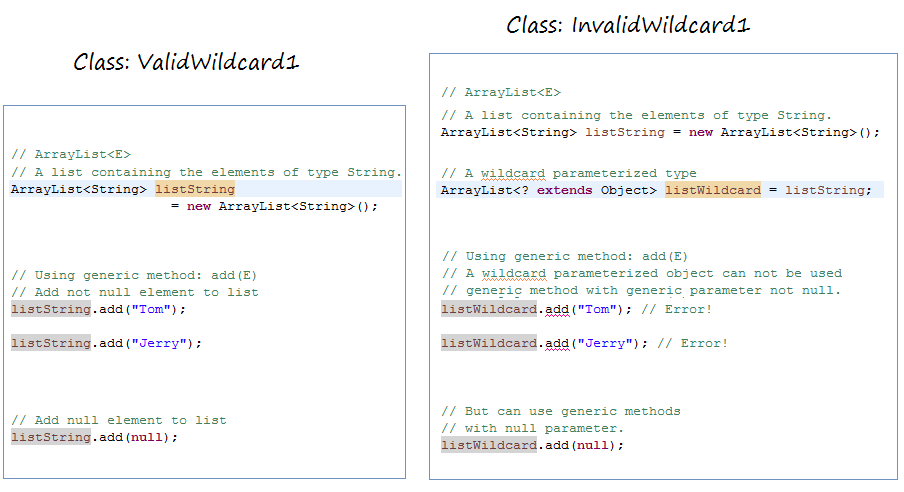ValidWildcard1.java
``````
package org.o7planning.tutorial.generics.w;

import java.util.ArrayList;

public class ValidWildcard1 {

public static void main(String[] args) {

// A list containing the elements of type String.
ArrayList<String> listString = new ArrayList<String>();

// Add not null element to list.

// Add null element to list.
}

}
``````
InvalidWildcard1.java
``````
package org.o7planning.tutorial.generics.w;

import java.util.ArrayList;

public class InvalidWildcard1 {

public static void main(String[] args) {

// A list with wildcard parameterized type.
ArrayList<? extends Object> listWildcard = listString;

// You can not use the 'add(E)' method
// with not null value of parameter.

// Add a null element to list.

}

}
``````

### 6.3- Wildcard can not participate in the new operator

A wildcard parameterized type is not a concrete type that could appear in a new expression. It just hints the rule enforced by java generics that which types are valid in any particular scenario where wild cards have been used.
``````
// Wildcard can not participate in the 'new' operator.
List<? extends Object> list= new ArrayList<? extends Object>();
``````

#### View more Tutorials:

Maybe you are interested

These are online courses outside the o7planning website that we introduced, which may include free or discounted courses.